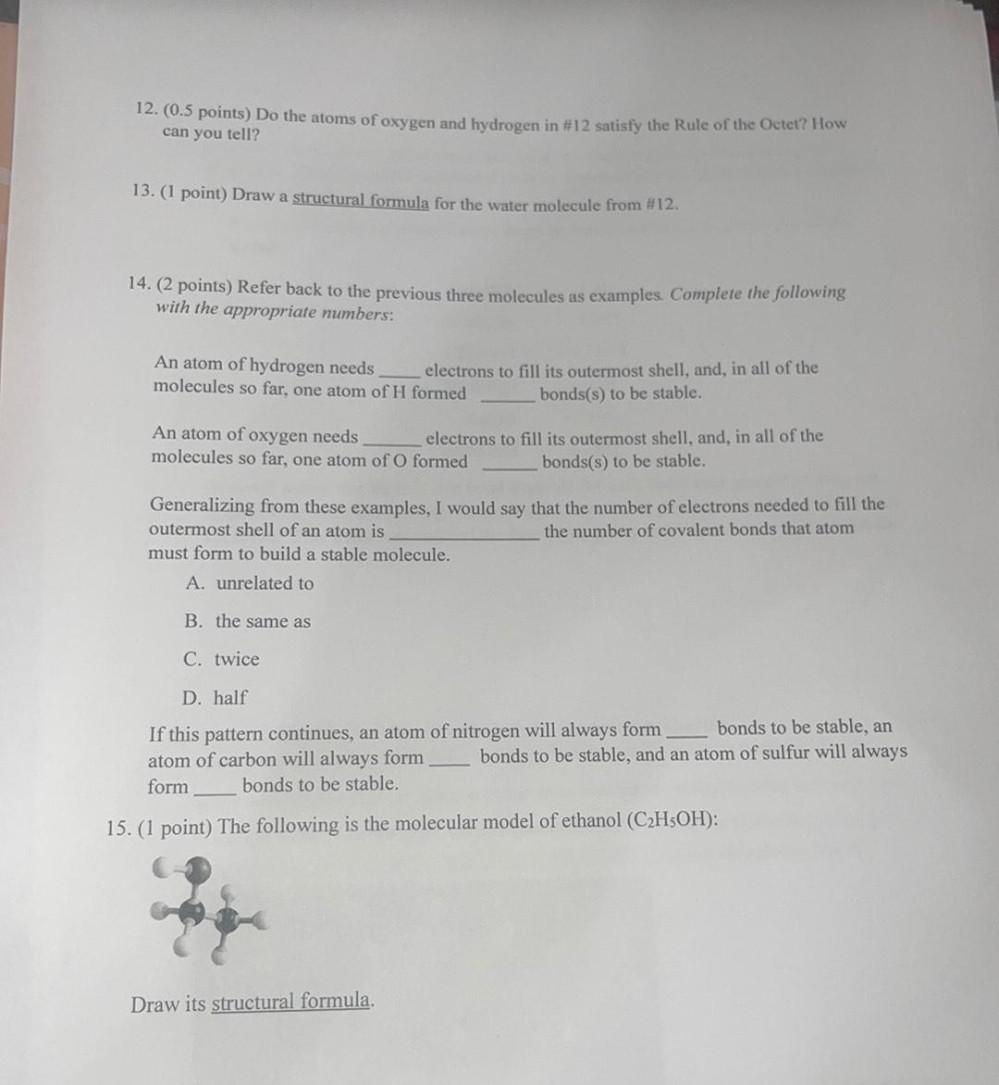Question:

# 12. (0.5 points) Do the atoms of oxygen and hydrogen in #12 satisfy the Rule of the Octet? How can you tell? 13. (1 point) Draw12. (0.5 points) Do the atoms of oxygen and hydrogen in #12 satisfy the Rule of the Octet? How can you tell? 13. (1 point) Draw a structural formula for the water molecule from #12. 14. (2 points) Refer back to the previous three molecules as examples. Complete the following with the appropriate numbers: An atom of hydrogen needs electrons to fill its outermost shell, and, in all of the molecules so far, one atom of H formed bonds(s) to be stable. An atom of oxygen needs electrons to fill its outermost shell, and, in all of the molecules so far, one atom of O formed bonds(s) to be stable. Generalizing from these examples, I would say that the number of electrons needed to fill the outermost shell of an atom is the number of covalent bonds that atom must form to build a stable molecule. A. unrelated to B. the same as C. twice D. half If this pattern continues, an atom of nitrogen will always form bonds to be stable, an atom of carbon will always form bonds to be stable, and an atom of sulfur will always form bonds to be stable. 15. (1 point) The following is the molecular model of ethanol (C2H5OH): Draw its structural formula.